Courses

# Test: Dipole Moment

## 19 Questions MCQ Test Chemistry Class 11 | Test: Dipole Moment

Description
This mock test of Test: Dipole Moment for Class 11 helps you for every Class 11 entrance exam. This contains 19 Multiple Choice Questions for Class 11 Test: Dipole Moment (mcq) to study with solutions a complete question bank. The solved questions answers in this Test: Dipole Moment quiz give you a good mix of easy questions and tough questions. Class 11 students definitely take this Test: Dipole Moment exercise for a better result in the exam. You can find other Test: Dipole Moment extra questions, long questions & short questions for Class 11 on EduRev as well by searching above.
QUESTION: 1

### Direction (Q. Nos. 1-12) This section contains 12 multiple choice questions. Each question has four choices (a), (b), (c) and (d), out of which ONLY ONE option is correct. Number of π bonds in the following structure is–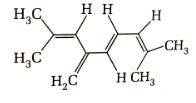Solution:

In a given structure there are 4 -pi bonds.Hence, total number of pi electrons are 8.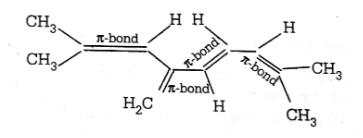QUESTION: 2

### Correct decreasing order of dipole moment of CH3F, CH3CI and CH3Br is

Solution: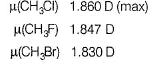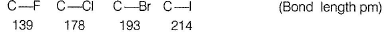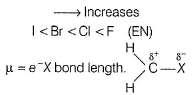Bond length and (EN) oppose each other, with the larger halogens having longer bonds but weaker (EN). Thus CH3CI > CH3F > CH3Br.

QUESTION: 3

### Consider the following compounds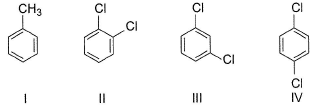Q. Increasing order of dipole moment of these compounds is

Solution: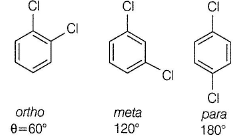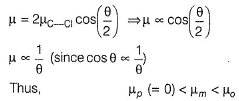Toluene with one —CH3 is less polar then m - and o-dichlorobenzene. Thus, IV < l < III < II.

QUESTION: 4

Dipole moment of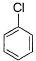is 1.5 D. Thus, dipole moment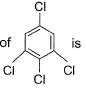Solution:

If one considers following molecule, it is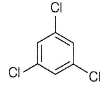Symmetrical and thus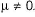In the given species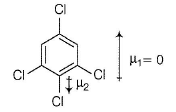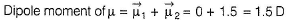QUESTION: 5

Given for HCI and HI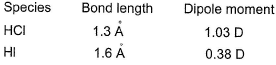Q. Ratio of fraction of electric charge existing on each atom in HCI and HI is

Solution: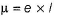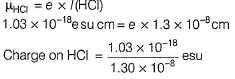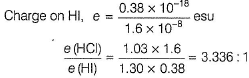QUESTION: 6

Ortho-isomers have dipole moments. In which cases dipole moments are maximum and minimum?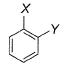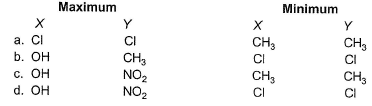Solution: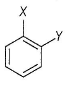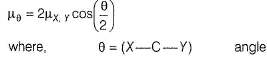Larger the value of θ, smaller the value of μ. Dipole-dipole repulsion (due to large groups (as Cl, Cl) increases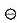, thus decreases p.
Intramolecular H-bonding (as OH and NO2) decreases θ, thus increases μ.

Repulsion
(Cl—Cl) > (OH—OH) > (CH3—CH3)
Thus, μ is minimum when X = V = Cl and μ is maximum when X = OH
Y = NO2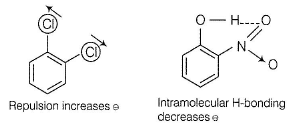QUESTION: 7

Which of the following is nonpolar but contains polar bonds?

Solution:

(EN) difference of Cl and H, makes Cl atom an electron withdrawing. Thus, HCI is polar in nature.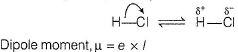QUESTION: 8

Bond length of H— Cl is 1.2476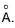Thus, its dipole moment is

Solution: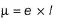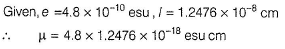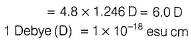QUESTION: 9

PCIx F5 - x shows non-polar behaviour when x is

Solution: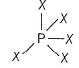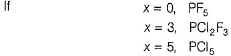QUESTION: 10

Which of the following bonds is the weakest?

Solution:

van der Waals` force is the weakest force of attraction and covalent bond is the strongest. Correct order is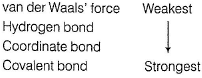QUESTION: 11

In which of the following sets of compounds dipole moment of I is larger than that of II?

Solution: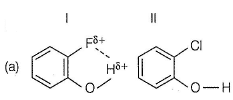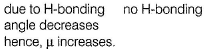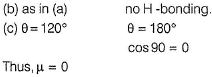QUESTION: 12

Which of the following would have a permanent dipole?

Solution: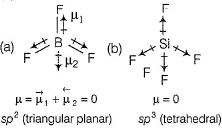(c) SF4 has one lone pair making it polar permanently.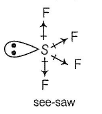sp3d-hybridised S-atom.
(d) XeF4 has two lone pairs in opposite side thus μ = 0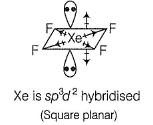*Multiple options can be correct
QUESTION: 13

Direction (Q. Nos. 13-15) This section contains 4 multiple choice questions. Each question has four choices (a), (b), (c) and (d), out of which ONE or  MORE THANT ONE  is correct.

Q. Which of the following has/have zero dipole moments?

Solution: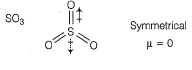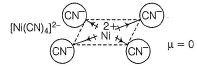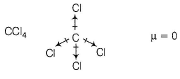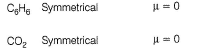CO, SO2, H2O, NH3 and HCI have polarity thus, have dipole moments.

*Multiple options can be correct
QUESTION: 14

Dipole moment is shown by

Solution: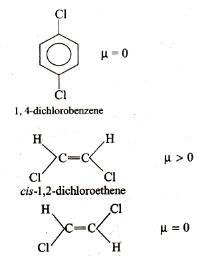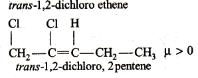*Multiple options can be correct
QUESTION: 15

The molecules that will have dipole moment are

Solution: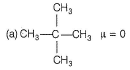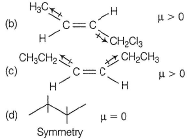QUESTION: 16

Direction (Q. Nos. 16-17) This section contains  a paragraph, each describing theory, experiments, data etc. three Questions related to paragraph have been given.Each question have only one correct answer among the four given options (a),(b),(c),(d).

CH4 is subjected to photochemical reaction and changes to CH3CI, CH2CI2, CHCI3 and CCI4.

Q.
Select the correct alternate.

Solution: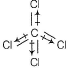Resultant dipole moment of CCI4 = 0.

QUESTION: 17

CH4 is subjected to photochemical reaction and changes to CH3CI, CH2CI2, CHCI3 and CCI4.

Q. One of the intermediates of the photochemical reaction is

Solution: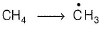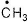has one unpaired electron in unhybridised p-orbital.
Thus, sp2-hybridised paramagnetic.

*Answer can only contain numeric values
QUESTION: 18

Direction (Q. Nos. 18 and 19) This section contains 2 questions. when worked out will result in an integer from 0 to 9 (both inclusive).

Q. How many of the following molecules are polar?

Cl2, ICI, BF3, NO, SO2
XeF4, CH2CI2, OCS, CO2

Solution:

Due to electronegativity difference between the bonded atoms. ICI, NO, SO2, CH2CI2, OCS are polar compounds.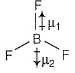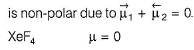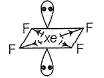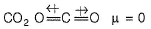*Answer can only contain numeric values
QUESTION: 19

How many molecules out of the following have non-zero value of dipole moments?

CH2CI2, CH4, CCI4, H2O, CHCI3, p-dichloro benzene, o-cresol, p-xylene, SCI2, BF3, IBr, HCHO

Solution:

CH2CI2,H2O,CHCI3,o-cresol, SCI2, IBr and HCHO (in all seven) have dipole moments.